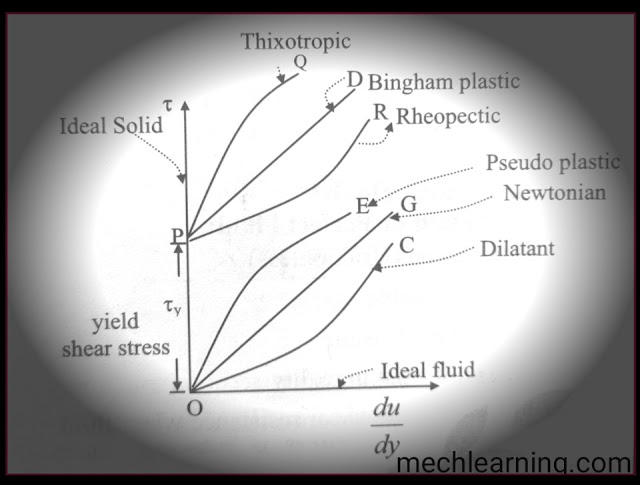# What is Fluid ?Types of fluids in fluid mechanics with definitions and examples

In a fluid mechanics on the fluid is a substance which deforms continuously when subjected to an external shear stress, called as fluid.

In a simple manner, Fluid defined as A substance, which has no fixed shape and are  able to flow with easily in called as fluid.

According to the behaviour of the fluid under the same atmospheric condition the fluid is having difference types of categories.

## Graphical representation of various types of fluids:-

The following image shows the relationship between shear stress and the velocity gradient for the various types of fluids.Types of fluids in fluid mechanics with definitions and examples

# Types of fluids:-

1. Ideal fluid or perfect fluid.
2. Real fluid.
3. Newtonian fluid.
4. Non-newtonian fluid.
5. Dilatant fluid.
6. Pseudo plastic fluid.
7. Bingham plastic fluid.
8. Thixotropic fluid.
9. Rheopectic fluid.
10. Viscoelastic fluid.
11. Compressible fluid.
12. Incompresible fluid.

#### 1. Ideal fluid or perfect fluid.Ideal or perfect fluid

A fluid which is incompresible and having no viscosity that is frictionless, Sach fluid called as ideal fluid or perfect fluid. And also Ideal fluid having no surface tension.
Ideal fluid having negligible friction.
The ideal fluid does not exist in reality.

#### 2. Real fluid.Real fluid
A fluid which having the properties such as viscosity, surface tension etc. Is called as real fluid.
Generally all the type of fluids are considered as a real fluids.

Examples:- Water

#### 3. Newtonian fluid.

A fluid which follows the Newton's law of viscosity is called as a newtonian fluids.
Newtonian fluid having constant viscosity and the viscosity is independent of the rate of deformation.

There will be linear relationship between shear stress and resulting rate of deformation.

Examples:- gasoline, Air, light oil.

#### 4. Non newtonian fluid

A fluid which does not follows the Newton's law of viscosity is called as non newtonian fluid.
The shear stress in non newtonian fluid is not directly proportional to rate of Shear strain or deformation.

The study of non newtonian fluid is called as rheology.

Examples:- Plastics, pastes etc.

#### 5. Dilatant fluid:-

A dilatant fluid, apparent viscosity is increases with the rate of deformation.
For given rate of the formation the shear stress are required increases with the time.
Because of that dilatant fluid is also called as shear thickening fluids.

Examples:- Butter, sand, sugar solution etc.

#### 6. Pseudo plastic fluid:-

A pseudo plastic fluid apparent viscosity decreases with the rate of deformation aur share rate.
For a given share rate the shear stress required is less than the time.
Because of that pseudo plastic fluid is also called as shear thinning fluids.

Examples:- blood, milk, paints, paper pulps etc.

#### 7. Bingham plastic fluid:-Bingham plastic fluid

The bingham plastic fluid is also called as ideal plastic fluid.
Some materials like toothpaste can resist of finite shear stress and thus behave as a solid but deform continuously when shear stress exceeds the yield stress.
And behave as a fluid in a linear relation between shear stress and rate of deformation.

Examples:- toothpaste, drilling muds, sewage sludge etc.

#### 8. Thixotropic fluid:-

Thixotropic fluid shows a decrease in rate of shear strain with the time under a constant applied shear stress.

Examples:- lipstick, printer ink etc.

#### 9. Rheopectic fluid:-Rheopectic fluid

The fluid which requires a gradually increasing shear stress to maintain a constant strain rate iscalled as Rheopectic fluid.

Examples:- Gypsum

#### 10. Viscoelastic fluid:-

When the applied shear stress is released, after that the formation of a fluid is partially return to their original shape such a fluid is called as viscoelastic fluid.

Examples:- combination of liquids and solids in a pipes, biological fluids etc.

#### 11. Compressible fluid:-

With the application of forces the density of fluid changes is called as compressible fluid.
The density of the compressible fluid is does not constant within given time.

Examples:- all gases.

#### 12. Incompressible fluid:-

With the application of forces the density of fluid does not changes is called as  incompressible fluid.
The density of incompressible fluid is constant with given time.

Examples:- all Liquids.

## Overview of graphical representation of types of fluids is as follows:-Types of fluids in fluid mechanics with definitions and examples

The x-axis represent the shear stress and y-axis represent the velocity gradient.
This graph gives relationship between shear stress and the velocity gradient.

For the newtonian fluid shear stress is directly proportional to velocity gradient. This called Newton's law of viscosity.
For the non newtonian fluid shear stress is is not directly proportional to the velocity gradient.
In simple word non newtonian fluid do not follow the Newton's law of viscosity.

Here we concluding, If you have any queries about the topic of Types of fluids in fluid mechanics with definitions and examples then feel free to comment below.

Related articles:

Previous Post
Next Post

#### 1 comment:

1.Harrah's Reno Casino & Hotel - Mapyro
MapYO is a fun and easy way to 경산 출장샵 find, recommend and talk about what's great 양산 출장마사지 and 순천 출장안마 not 제주 출장샵 so 광주 출장안마 great in Reno.

If you have any doubt, please let me know